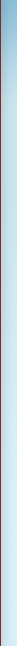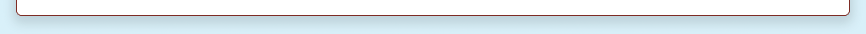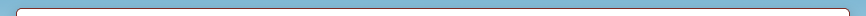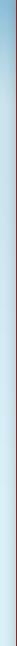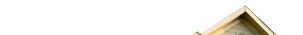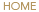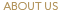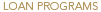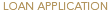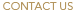Debt Service Calculator
Use this form to calculate the Debt Service Coverage Ratio (DSCR) of a proposed loan amount.

 Income \$ Expenses + Reserves \$ Requested Loan Amount \$ Amortization Interest Rate % Results NOI \$ 0 DSCR 0Commercial Calculators

Max Loan Amount Calculator
Calculate the max loan amount that a property will qualify for.

Residential Calculators

Payment Calculator
Calculate Payments on a traditional mortgage.

Interest Only Payment Calculator
Calculate your payments with an interest only loan.

2nd Mortgage Calculator

Affordability Calculator
How much house / monthly payment can you afford?

Mortgage Amortization Calculator
Detailed breakdown of principal and interest payments over the life of your loan.

Option ARM Calculator
Calculate four monthly payment options with an adjustable interest rate.

HELOC Calculator
Calculate payments, interest, and savings for a HELOC.

Blended Interest Rate Calculator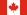Canadian Mortgage Calculator